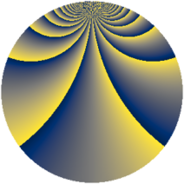# Properties

 Label 1840.2.bwLevel $1840$ Weight $2$ Character orbit 1840.bw Rep. character $\chi_{1840}(111,\cdot)$ Character field $\Q(\zeta_{22})$ Dimension $480$ Sturm bound $576$

# Related objects

## Defining parameters

 Level: $$N$$ $$=$$ $$1840 = 2^{4} \cdot 5 \cdot 23$$ Weight: $$k$$ $$=$$ $$2$$ Character orbit: $$[\chi]$$ $$=$$ 1840.bw (of order $$22$$ and degree $$10$$) Character conductor: $$\operatorname{cond}(\chi)$$ $$=$$ $$92$$ Character field: $$\Q(\zeta_{22})$$ Sturm bound: $$576$$

## Dimensions

The following table gives the dimensions of various subspaces of $$M_{2}(1840, [\chi])$$.

Total New Old
Modular forms 3000 480 2520
Cusp forms 2760 480 2280
Eisenstein series 240 0 240

## Trace form

 $$480q + 48q^{9} + O(q^{10})$$ $$480q + 48q^{9} + 48q^{25} - 24q^{29} + 24q^{41} - 24q^{49} - 60q^{69} + 96q^{77} - 72q^{81} + 264q^{89} - 96q^{93} + 264q^{97} + O(q^{100})$$

## Decomposition of $$S_{2}^{\mathrm{new}}(1840, [\chi])$$ into newform subspaces

The newforms in this space have not yet been added to the LMFDB.

## Decomposition of $$S_{2}^{\mathrm{old}}(1840, [\chi])$$ into lower level spaces

$$S_{2}^{\mathrm{old}}(1840, [\chi]) \cong$$ $$S_{2}^{\mathrm{new}}(92, [\chi])$$$$^{\oplus 6}$$$$\oplus$$$$S_{2}^{\mathrm{new}}(368, [\chi])$$$$^{\oplus 2}$$$$\oplus$$$$S_{2}^{\mathrm{new}}(460, [\chi])$$$$^{\oplus 3}$$